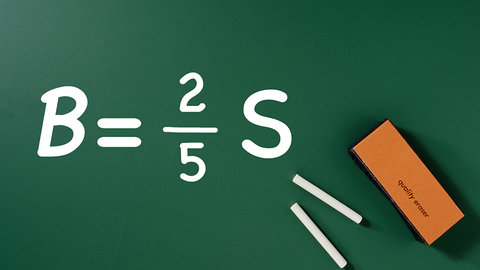Variables and Algebraic Expressions Episode 4 of Algebra I

The Great CoursesRelated videos

Operations and Expressions
Episode 5 of Algebra I
Discover that by following basic rules on how to treat coefficients and exponents, you can reduce very complicated algebraic expressions to much simpler ones. You start by using the commutative property of multiplication to rearrange the terms of an expression, making combining them relatively easy.
Rational Expressions, Part 1
Episode 28 of Algebra I
When one polynomial is divided by another, the result is called a rational function because it is the ratio of two polynomials. These functions play an important role in algebra. Learn how to add and subtract rational functions by first finding their common divisor.
Rational Expressions, Part 2
Episode 29 of Algebra I
Continuing your exploration of rational expressions, try your hand at multiplying and dividing them. The key to solving these complicated-looking equations is to proceed one step at a time. Close the lesson with a problem that brings together all you've learned about rational functions.
Episode 32 of Algebra I
Anytime you see a root symbol - for example, the symbol for a square root - then you're dealing with what mathematicians call a radical. Learn how to simplify radical expressions and perform operations on them, such as multiplication, division, addition, and subtraction, as well as combinations of these operations.
Quadratic Equations - Completing the Square
Episode 22 of Algebra I
After learning the definition of a function, investigate an additional approach to solving quadratic equations: completing the square. This technique is very useful when rewriting the equation of a quadratic function in such a way that the graph of the function is easily sketched.
An Introduction to the Course
Episode 1 of Algebra I
Professor Sellers introduces the general topics and themes for the course, describing his approach and recommending a strategy for making the best use of the lessons and supplementary workbook. Warm up with some simple problems that demonstrate signed numbers and operations.
Order of Operations
Episode 2 of Algebra I
The order in which you do simple operations of arithmetic can make a big difference. Learn how to solve problems that combine adding, subtracting, multiplying, and dividing, as well as raising numbers to various powers. These same concepts also apply when you need to simplify algebraic expressions, making it critical…
Percents, Decimals, and Fractions
Episode 3 of Algebra I
Continue your study of math fundamentals by exploring various procedures for converting between percents, decimals, and fractions. Professor Sellers notes that it helps to see these procedures as ways of presenting the same information in different forms.
Principles of Graphing in 2 Dimensions
Episode 6 of Algebra I
Using graph paper and pencil, begin your exploration of the coordinate plane, also known as the Cartesian plane. Learn how to plot points in the four quadrants of the plane, how to choose a scale for labeling the x and y axes, and how to graph a linear equation.
Solving Linear Equations, Part 1
Episode 7 of Algebra I
In this lesson, work through simple one- and two-step linear equations, learning how to isolate the variable by different operations. Professor Sellers also presents a word problem involving a two-step equation and gives tips for how to solve it.
Solving Linear Equations, Part 2
Episode 8 of Algebra I
Investigating more complicated examples of linear equations, learn that linear equations fall into three categories. First, the equation might have exactly one solution. Second, it might have no solutions at all. Third, it might be an identity, which means every number is a solution.
Slope of a Line
Episode 9 of Algebra I
Explore the concept of slope, which for a given straight line is its rate of change, defined as the rise over run. Learn the formula for calculating slope with coordinates only, and what it means to have a positive, negative, and undefined slope.